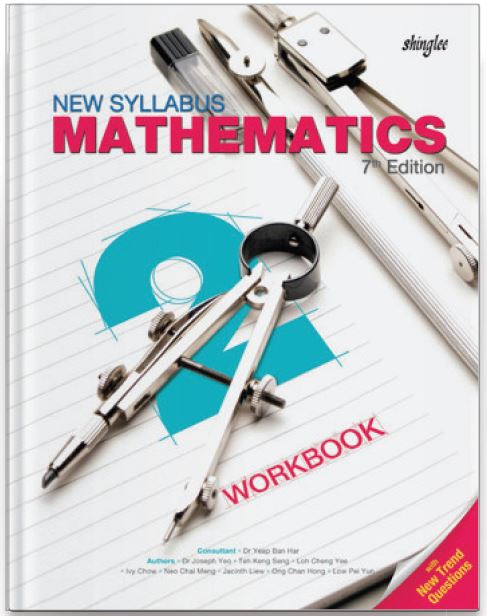Our prices are denominated in USD.

We ship internationally!# New Syllabus Mathematics Workbook 2 (7th Ed)

US\$11.25

New Syllabus Mathematics is the most widely-adopted series of secondary mathematics textbooks in Singapore. Following the three-part lesson approach, pupils begin with an anchor task, followed by a guided practice, before concluding with an independent practice. New concepts are introduced and built upon in the textbooks. Well-structured exercises developed in
accordance to the lesson objectives are found in the workbooks.

This is a 4-year course in preparation for Cambridge O-level.

This is a 4-year course.

For Secondary 2/ Grade 8 / Year 8

1. Direct and Inverse Proportions
Algebraic and Graphical Representations

2. Linear Graphs and Simultaneous Linear Equations
Horizontal and Vertical Lines, Solving Using Graphical and Algebraic Methods

3. Expansion and Factorisation of Quadratic Expressions

4. Further Expansion and Factorisation of Algebraic Expressions
Special Algebraic Identities

Solving and Applications

6. Algebraic Fractions and Formulae
Four Operations and Manipulation

7. Congruence and Similarity
Enlargement and Scale Drawings

8. Pythagoras' Theorem
Converse and Applications

9. Trigonometric Ratios
Right-Angled Triangles

10. Volume and Surface Area of Pyramids, Cones and Spheres
including Composite Figures

11. Probability of Single Events

12. Statistical Diagrams
Dot, Stem-and-Leaf Diagrams and Histograms for Grouped and Ungrouped Data

13. Averages of Statistical Data
Mean, Median and Mode